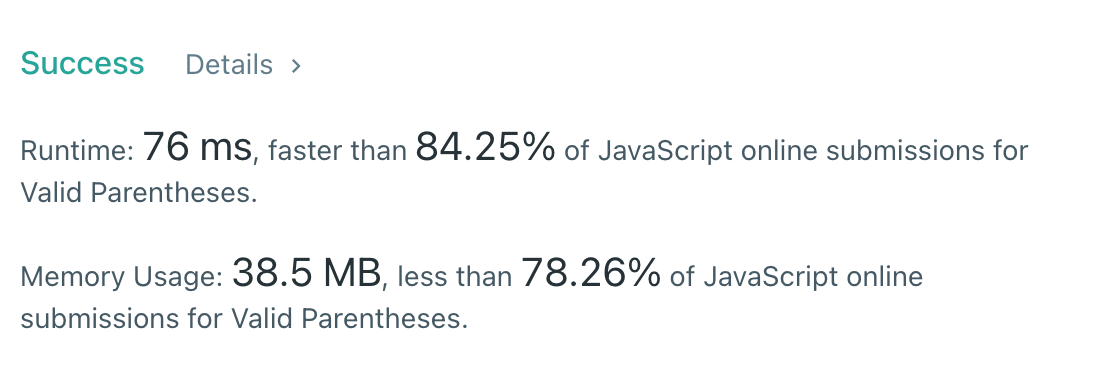# JavaScript Algorithms: Valid Parentheses (LeetCode)Given a string `s` containing just the characters `'('`, `')'`, `'{'`, `'}'`, `'['` and `']'`, determine if the input string is valid.

An input string is valid if:

1. Open brackets must be closed by the same type of brackets.

2. Open brackets must be closed in the correct order.

Example 1:

``````Input: s = "()"
Output: true
``````

Example 2:

``````Input: s = "()[]{}"
Output: true
``````

Example 3:

``````Input: s = "(]"
Output: false
``````

Example 4:

``````Input: s = "([)]"
Output: false
``````

Example 5:

``````Input: s = "{[]}"
Output: true
``````

Constraints:

• `1 <= s.length <= 104`
• `s` consists of parentheses only `'()[]{}'`.

### Solution

The best data structure here is `stack`. Because we need to check the right order of these parentheses. For example, if we have `{][}` the number of parentheses is correct, but the order is not.

So. the algorithm is pretty straightforward - go through these parentheses and if we see an opening character we need to push it to `stack`, if not (closing) - we need to check whether the top of the `stack` is a corresponding opening character.

Let's look at the example below `{[()]}`

``````iterations:

1) { // stack = ['{']
2) [ // stack = ['{', '[']
3) ( // stack = ['{', '[', '(']
4) ) // stack = ['{', '['] - we've matched closing ) to the latest item in stack (
5) ] // stack = ['{'] - we've matched closing ] to the latest item in stack [
6) } // stack = [] - we've matched closing } to the latest item in stack {
``````
``````const isValid = (s) => {
const stack = [];

for (let i = 0; i < s.length; i += 1) {
const top = stack[stack.length - 1];
if (s[i] === "(" || s[i] === "{" || s[i] === "[") {
stack.push(s[i]);
} else if (s[i] === ")" && top === "(" && stack.length !== 0) {
stack.pop();
} else if (s[i] === "]" && top === "[" && stack.length !== 0) {
stack.pop();
} else if (s[i] === "}" && top === "{" && stack.length !== 0) {
stack.pop();
} else {
return false;
}
}

return stack.length === 0;
};
``````

Let’s think about how we can optimize this solution.Photo by Jason Strull on Unsplash

We can create a `Set` with opening characters and `Map` with closing to opening key-value pairs. Now, we can easily understand if it's an opening one or not. And if not we can easily figure out whether the `stack` has the corresponding top with the opening character.

``````const isValid = (s) => {
const stack = [];
const openSet = new Set(["(", "{", "["]);
const closeOpenMap = new Map([
[")", "("],
["}", "{"],
["]", "["],
]);

for (let i = 0; i < s.length; i += 1) {
if (openSet.has(s[i])) {
stack.push(s[i]);
} else {
const pop = stack.pop();
if (pop !== closeOpenMap.get(s[i])) {
return false;
}
}
}

return stack.length === 0;
};
``````

It looks better, but we can still improve it.

Instead of pushing opening characters, we can push closing characters. And when we see some closing characters we can match it with the top of the `stack`. Thus we can reduce our codebase (use only `Map`).

Let's look at the same example below `{[()]}`

``````iterations:

1) { // stack = ['}']
2) [ // stack = ['}', ']']
3) ( // stack = ['}', ']', ')']
4) ) // stack = ['}', ']'] - we've matched closing ) to the latest item in stack (
5) ] // stack = ['}'] - we've matched closing ] to the latest item in stack [
6) } // stack = [] - we've matched closing } to the latest item in stack {
``````

Let’s implement this approach:

``````const isValid = (s) => {
const stack = [];
const map = new Map([
["(", ")"],
["[", "]"],
["{", "}"],
]);

for (let i = 0; i < s.length; i += 1) {
if (map.has(s[i])) {
stack.push(map.get(s[i]));
} else if (s[i] !== stack.pop()) {
return false;
}
}

return stack.length === 0;
};
``````

And we can check if the `s.length` is even or not. If not we can return false.

``````const isValid = (s) => {
if (s.length % 2 !== 0) return false;

const stack = [];
const map = new Map([
["(", ")"],
["[", "]"],
["{", "}"],
]);

for (let i = 0; i < s.length; i += 1) {
if (map.has(s[i])) {
stack.push(map.get(s[i]));
} else if (s[i] !== stack.pop()) {
return false;
}
}
return stack.length === 0;
};
``````

Now, I think it's optimal. So, the time complexity is `O(n)` and space complexity is `O(n)` as well because in the worst-case scenario if we get a sequence with only opening characters `(({[([{{[(` we'll push all of them to the `stack`.I hope, it was useful for you. Thanks for reading! Looking forward to your feedback. See you soon ✌️